# Visualising FFTs with FFTrees

## Visualizing FFTrees

The FFTrees package makes it very easy to visualize and evaluate fast-and-frugal trees (FFTs):

• Use the main FFTrees() function to create a set of FFTs (e.g., as an R object x of type FFTrees).

• Visualize a tree in x by evaluating plot(x).

The two key arguments for plotting are what and tree: Whereas the tree argument allows selecting between different trees in x (using tree = 1 by default), the what argument distinguishes between five main types of plots:

1. plot(x, what = 'all') visualizes a tree and corresponding performance statistics. This is also the default when evaluating plot(x).

2. plot(x, what = 'tree') visualizes only the tree diagram of the selected tree (without performance statistics).

3. plot(x, what = 'icontree') visualizes the tree diagram of the selected tree with icon arrays on exit nodes (with additional options for show.iconguide and n.per.icon.

4. plot(x, what = 'cues') visualizes the current cue accuracies in ROC space (by calling the showcues() function).

5. plot(x, what = 'roc') visualizes a performance comparison of FFTs and competing algorithms in ROC space.

The other arguments of the plot.FFTrees() function allow further customization of the plot (e.g., by defining labels and parameters, or selectively hiding or showing elements).

In the following, we illustrate both ways by creating FFTs based on the titanic data (included in the FFTrees package).

### The Titanic data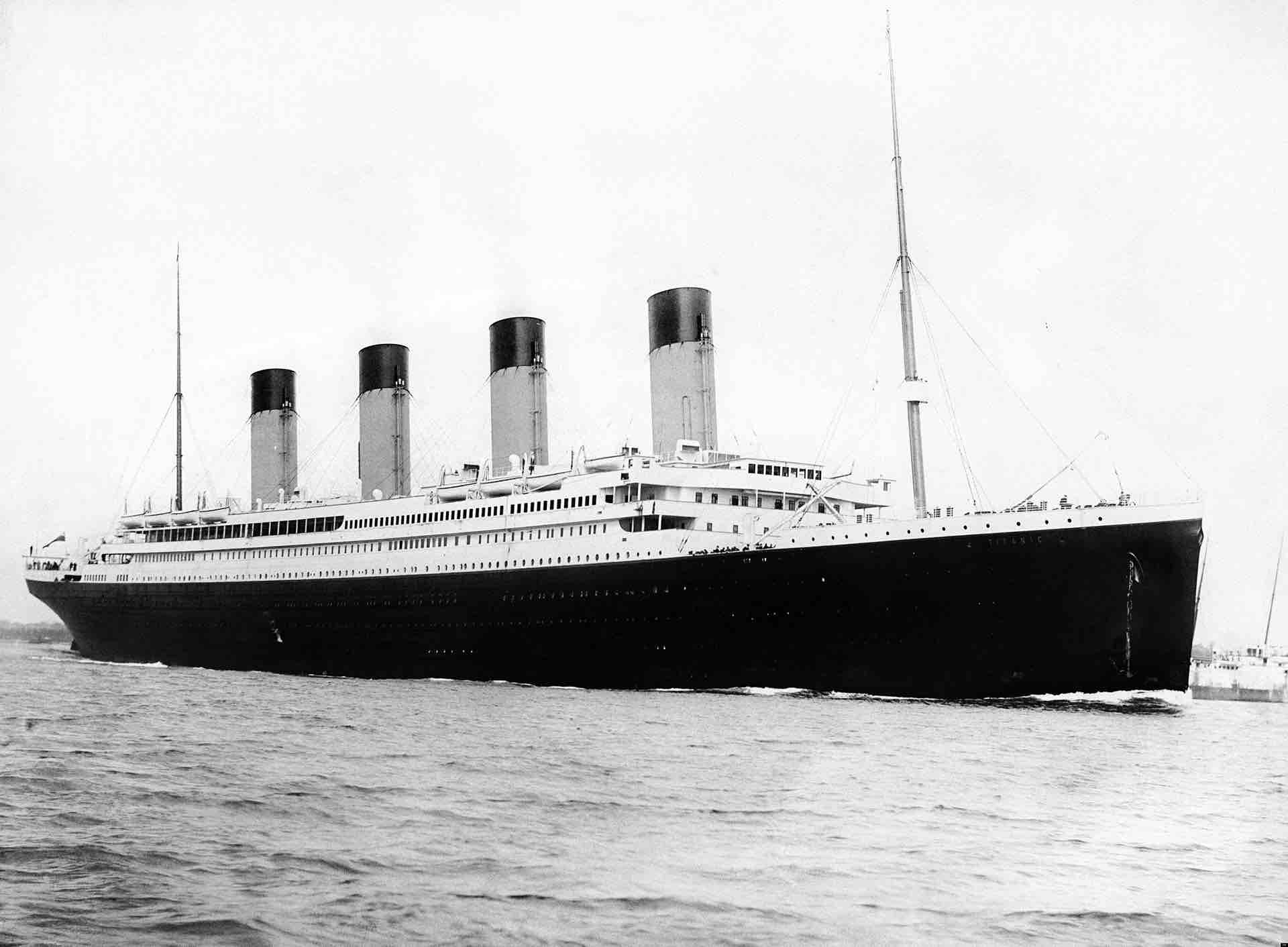The titanic dataset contains basic survival statistics of Titanic passengers. For each passenger, we know in which class s/he traveled, as well as binary categories specifying age, sex, and survival information. To get a first impression, we inspect a random sample of cases:

set.seed(12)  # reproducible randomness
rcases <- sort(sample(1:nrow(titanic), 10))

# Sample of data:
knitr::kable(titanic[rcases, ], caption = "A sample of 10 observations from the titanic data.")
A sample of 10 observations from the titanic data.
class age sex survived
1271 third child male FALSE

Our current goal is to fit FFTs to this dataset. This essentially asks:

• Can we use the information in the cues class, age and sex to decide whether a passenger survived?

First, let’s create an FFTrees object (called titanic.fft) from the titanic dataset:

# Create FFTs for the titanic data:
titanic.fft <- FFTrees(formula = survived ~.,
data = titanic,
main = "Surviving the Titanic",
decision.labels = c("Died", "Survived"))       

Note that we used the entire titanic data (i.e., all 2201 cases) to train titanic.fft, rather than specifying train.p to set aside some proportion of it or specifying a dedicated data.test set for predictive purposes. This implies that our present goal is to fit FFTs to the historic data, rather than on create and use FFTs to predict new cases.

### Visualising cue accuracies

We can visualize individual cue accuracies (specifically their sensitivities and specificities) by including the what = 'cues' argument within the plot() function. Let’s apply the function to the titanic.fft object to see how accurate each of the cues were on their own in predicting survival:

plot(titanic.fft, what = "cues", main = "Cues predicting Titanic survival")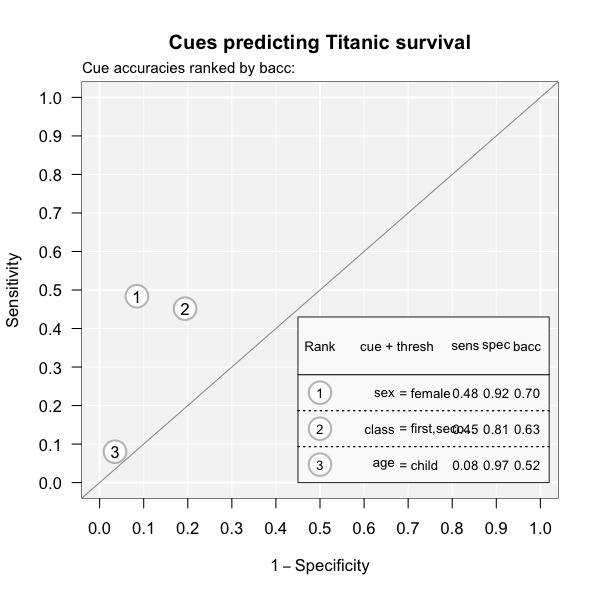Figure 1: Cue accuracies of FFTs predicting survival in the titanic dataset.

Given the axes of this plot, good performing cues should be near the top left corner of the graph (i.e., exhibit both a low false alarm rate and a high hit rate). For the titanic data, this implies that none of the cues predicts very well on its own. The best individual cue appears to be sex (indicated as 1), followed by class (2). By contrast, age (3) seems a pretty poor cue for predicting survival on its own (despite its specificity of 97%).

Inspecting cue accuracies can provide valuable information for constructing FFTs. While they provide lower bounds on the performance of trees (as combining cues is only worthwhile when this yields a benefit), even poor individual cues can shine in combination with other predictors.

### Visualizing FFTs and their performance

To visualize the tree from an FFTrees object, use plot(). Let’s plot one of the trees (Tree #1, i.e., the best one, given our current goal):

plot(titanic.fft, tree = 1)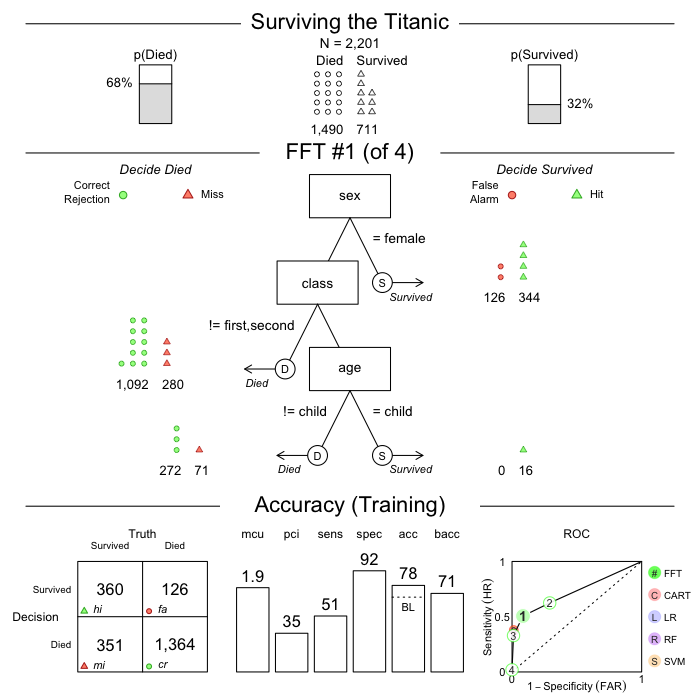Figure 2: Plotting the best FFT of an FFTrees object.

The resulting plot visualizes one out of rtitanic.fft$$trees$$n\ possible trees in thetitanic.fftobject. Astree = 1corresponds to the best tree given our currentgoalfor selecting FFTs, we could have plotted the same tree by specifyingtree = ‘best.train’.

As Figure 2 contains a lot of information in three distinct panels, let’s briefly consider their contents:

1. Basic dataset information: The top row of the plot shows basic information on the current dataset: Its population size (N) and the baseline frequencies of the two categories of the criterion variable.

2. FFT and classification performance: The middle row shows the tree (in the center) as well as how many cases (here: persons) were classified at each level in the tree (on either side). For example, the current tree (Tree #1 of 4) can be understood as:

• If a person is female, decide that they survived.
• Otherwise, if a person is neither in first nor in second class, decide that they died.
• Finally, if the person is a child, predict they survived, otherwise decide that they died.
3. Accuracy and performance information: The bottom row shows general performance statistics of the FFT:
As our models in titanic.fft were trained on the entire titanic dataset, we fitted FFTs to its 2201 cases, rather than setting aside some data for predictive purposes. The panel label reflects this important distinction:

• If the results of fitting data (i.e., data used to build the tree) are displayed, we’ll see a “Training” label.

• If a testing dataset separate from the one used to build the tree is used, we’ll see a “Prediction” label.

The bottom panel provides performance information and is structured into three subpanels:

1. The classification table (on the left) shows the relationship between the true criterion states (as columns) and predicted decisions (as rows). The abbreviations hi (hits) and cr (Correct rejections) denote correct decisions; mi (misses) and fa (false-alarms) denote incorrect decisions.

2. A range of vertical levels (in the middle) show the tree’s cumulative performance in terms of two frugality measures (mcu and pci) and various accuracy measures (sensitivity, specificity, accuracy, and balanced accuracy (see Accuracy statistics for details).

3. Finally, the plot (on the right) shows an ROC curve comparing the performance of all trees in the FFTrees object. Additionally, the performance of logistic regression (blue) and CART (red) are shown. The tree plotted in the middle panel is highlighted in a solid green color (i.e., Figure 2 shows Tree #1).

Specifying additional arguments of plot() changes what and how various elements are being displayed.

• what should be visualized? The what argument selects contents to be plotted:

• When what = 'all' (as by default), the plot shows both a tree diagram and a range of corresponding performance statistics. Using one of the other what options narrows the range of what is being shown:

• To only visualize a bare tree diagram (without performance statistics), we specify what = "tree" (formerly stats = FALSE).

• To visualize the tree diagram with icon arrays on exit nodes, we specify what = "icontree" (with additional options for show.iconguide and n.per.icon).

• To visualize the performance comparison (for different FFTs and competing algorithms) in ROC space, we specify what = "roc".

The following examples illustrate the wide range of corresponding plots:

# Plot tree diagram with icon arrays:
plot(titanic.fft, what = "icontree",
n.per.icon = 50, show.iconguide = TRUE)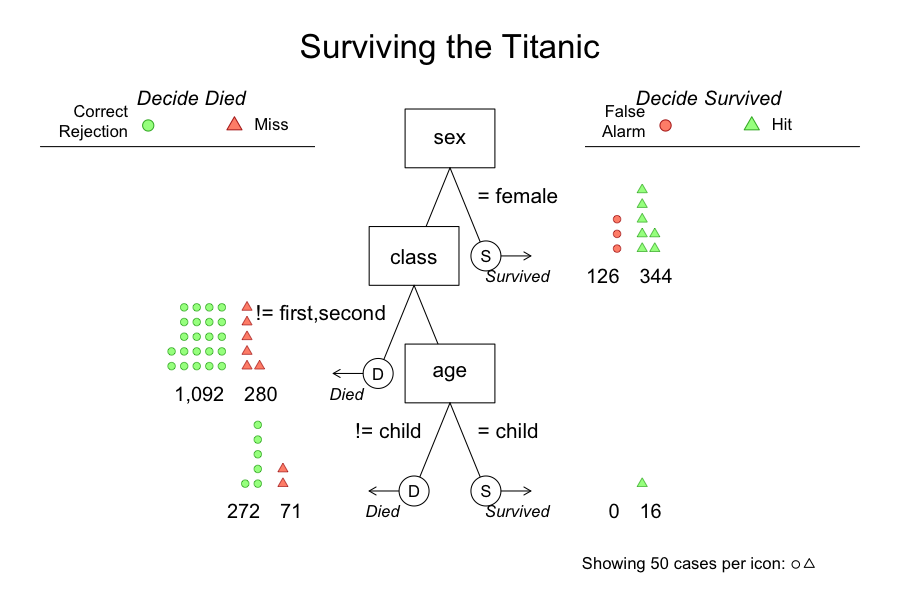Figure 3: An FFT diagram with icon arrays on exit nodes.

# Plot only the performance comparison in ROC space:
plot(titanic.fft, what = "roc")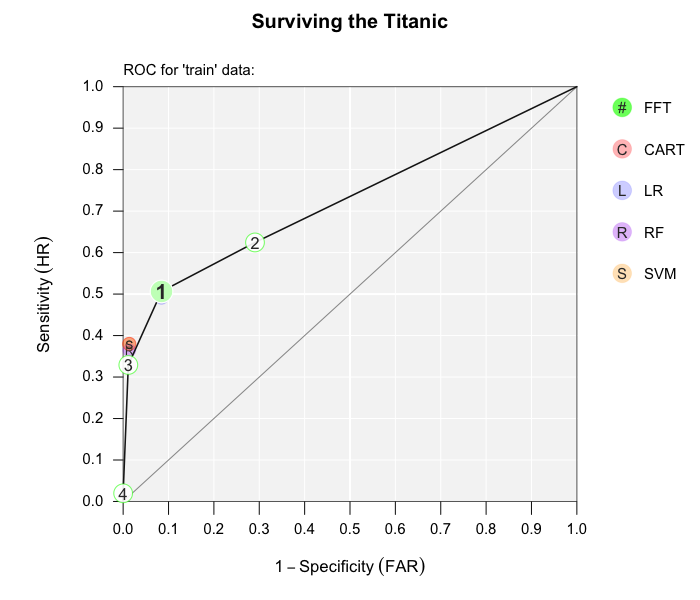Figure 4: Performance comparison of FFTs in ROC space.

• When the main options for what do not suffice, various show. arguments (i.e., show.header, show.tree, show.confusion, show.levels, show.roc, show.icons, and show.iconguide) allow to selectively turn on or turn off specific elements of the overall plot. For example:
# Hide some elements of the FFT plot:
plot(titanic.fft,
show.icons = FALSE,     # hide icons
show.iconguide = FALSE, # hide icon guide
)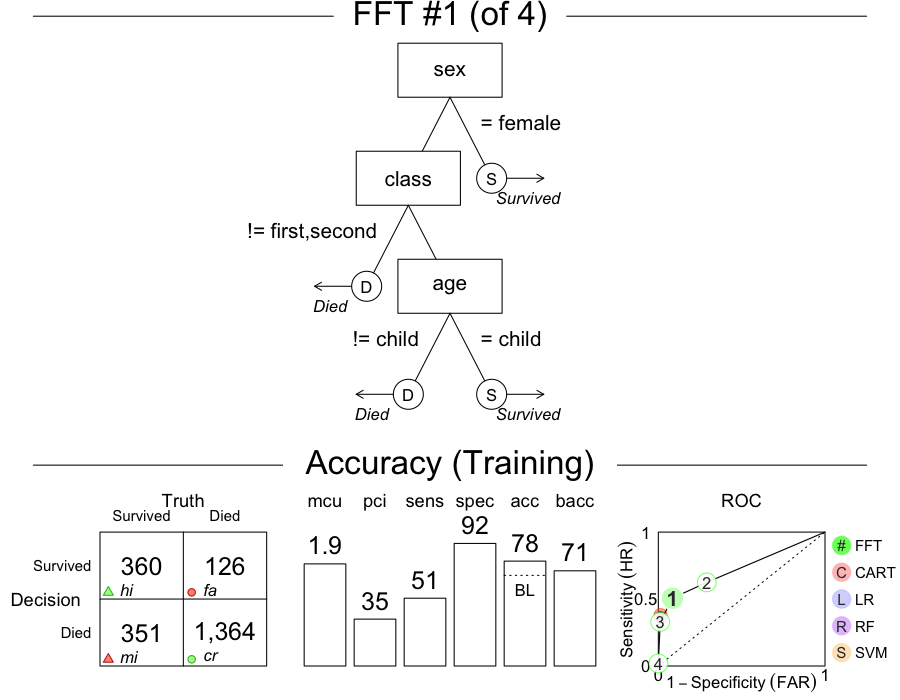Figure 5: Plotting selected elements.

• tree: Which tree do we want to plot? As FFTrees objects typically contain multiple FFTs, we need to indicate which tree we want to visualize. We usually specify the tree to show by an integer value, such as tree = 2, which will plot the corresponding tree (i.e., Tree #2) of the FFTrees object. Alternatively, we can specify tree = "best.train" or tree = "best.test" to visualize the best training or prediction tree, respectively. This selects and shows the tree with the highest goal value (e.g., weighted accuracy wacc) when fitting or testing data.

• data: Which data do we want to apply the tree to? We can specify data = "train" or data = "test" to distinguish between a training and testing dataset (if available) in the FFTrees object. As not all FFTrees objects contain test data, data is set to data = "train" by default.

As the data and tree arguments can both refer to datasets used for training or fitting (i.e., the “train” or “test” sets), they should be specified consistently. For instance, the following command would visualize the best training tree in titanic.fft:

plot(titanic.fft, tree = "best.train")

as data = "train" by default. However, the following analog expression would fail:

plot(titanic.fft, tree = "best.test")

for two distinct reasons:

1. When data remains unspecified, its default is data = "train". Thus, asking for tree = "best.test" would require switching to data = "test".

2. More crucially, titanic.fft was created without any test data. Hence, asking for the best test tree does not make sense — which is why plot() will show the best training tree (with a warning).

### Plotting performance for new data

Shifting our emphasis from fitting to prediction, we primarily need to specify some test data that was not used to train the FFTrees object. When predicting performance for a new dataset (e.g.; data = test.data), the plotting and printing functions will automatically apply an existing FFTrees object to the new data and compute corresponding performance statistics (using the fftrees_apply() function). However, when applying existing FFTs to new data, the changes to the FFTrees object are not stored in the input object, unless the (invisible) output of plot.FFTrees() or print.FFTrees() is re-assigned to that object. The best way to fit FFTs to training data and evaluate them to test data is to explicitly include both datasets in the original FFTrees() command by either using its data.test or its train.p argument.

For example, we can repeat the previous analysis, but now let’s create separate training and test datasets by including the train.p = .50 argument. This will split the dataset into a 50% training set, and a distinct 50% testing set. (Alternatively, we could specify a dedicated test data set by using the data.test argument.)

set.seed(100)  # for replicability of the training/test split
titanic.pred.fft <- FFTrees(formula = survived ~.,
data = titanic,
train.p = .50,  # use 50% to train, 50% to test
main = "Titanic",
decision.labels = c("Died", "Survived")
)

Here is the best training tree applied to the training data:

# print(titanic.pred.fft, tree = 1)
plot(titanic.pred.fft, tree = 1)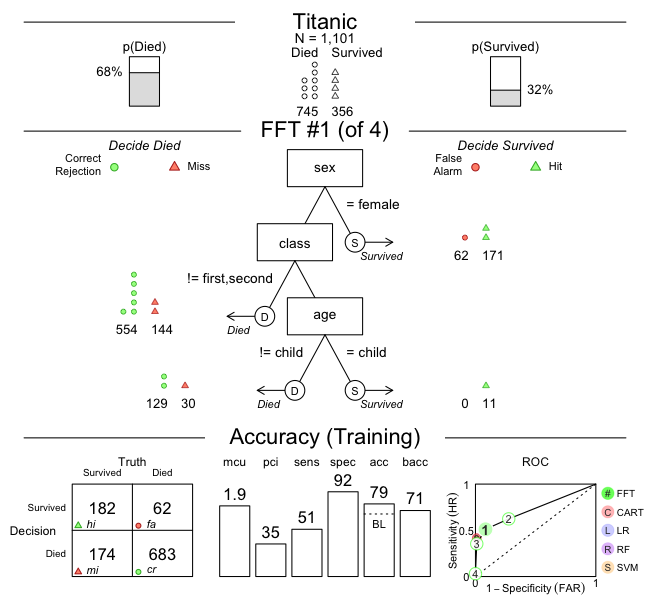Figure 6: Plotting the best FFT on training data.

Tree #1 is the best training tree — and could also be visualized by plot(titanic.pred.fft, tree = "best.train"). This tree has a high specificity of 92%, but a much lower sensitivity of just 53%. The overall accuracy of the tree’s classifications is at 79%, which exceeds the baseline, but is far from perfect. However, as we can see in the ROC table, a logistic regression (LR) would not perform much better, and CART performed even worse than Tree #1.

Now let’s inspect the performance of the same tree on the test data:

# print(titanic.pred.fft, data = "test", tree = 1)
plot(titanic.pred.fft, data = "test", tree = 1)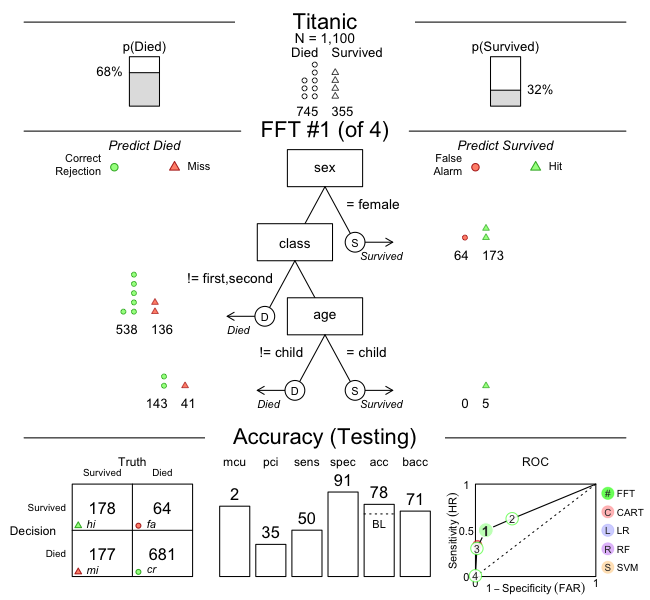Figure 7: Plotting the best FFT on test data.

We could have visualized the same tree by asking for plot(titanic.pred.fft, data = "test", tree = "best.test"). Note that the label of the bottom panel has now switched from “Accuracy (Training)” to “Accuracy (Testing)”. Both the sensitivity and specificity values have decreased somewhat, which is typical when using a model (fitted on training data) for predicting new (test) data.

Let’s visualize the prediction performance of Tree #2, the most liberal tree (i.e., with the highest sensitivity):

plot(titanic.pred.fft, data = "test", tree = 2)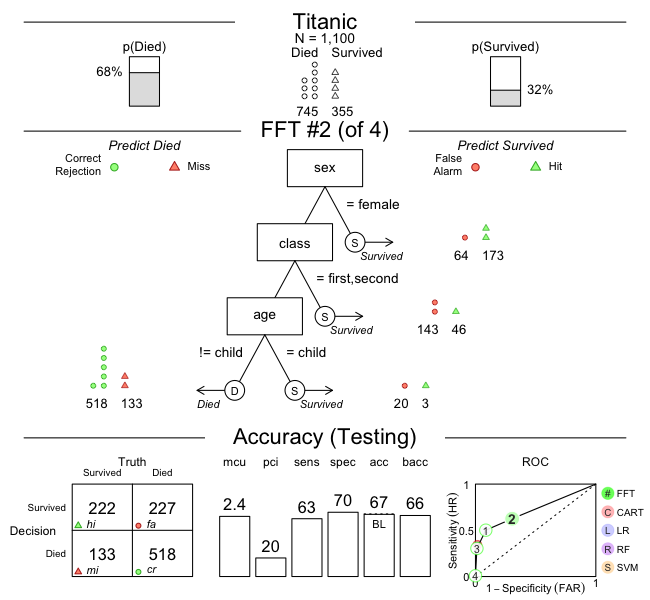Figure 8: Plotting Tree #2.

This alternative tree has a better sensitivity (of 63%), but its overall accuracy decreased to about baseline level (of 67%).

Whereas comparing training with test performance illustrates the trade-offs between mere fitting and genuine predictive modeling, comparing the performance details of various FFTs illustrates the typical trade-offs that any model for solving binary classification problems engages in. Importantly, both types of trade-offs are rendered transparent when using FFTrees.

## Vignettes

Here is a complete list of the vignettes available in the FFTrees package:

Vignette Description
Main guide: FFTrees overview An overview of the FFTrees package
1 Tutorial: FFTs for heart disease An example of using FFTrees() to model heart disease diagnosis
2 Accuracy statistics Definitions of accuracy statistics used throughout the package
3 Creating FFTs with FFTrees() Details on the main function FFTrees()
4 Manually specifying FFTs How to directly create FFTs with my.tree without using the built-in algorithms
5 Visualizing FFTs with plot() Plotting FFTrees` objects, from full trees to icon arrays
6 Examples of FFTs Examples of FFTs from different datasets contained in the package